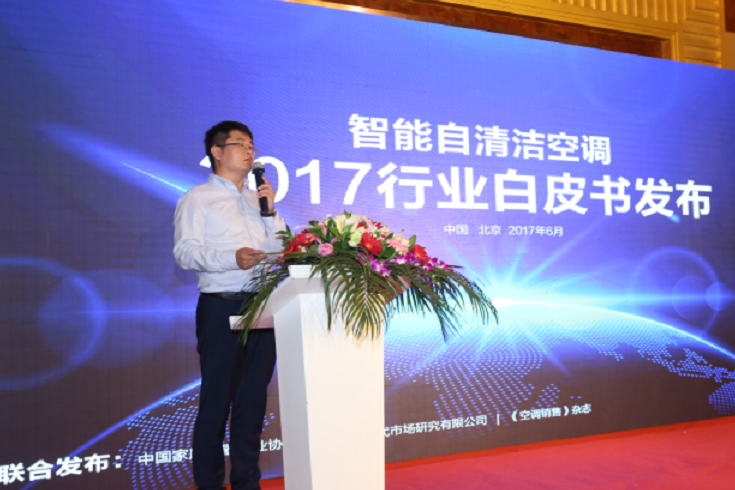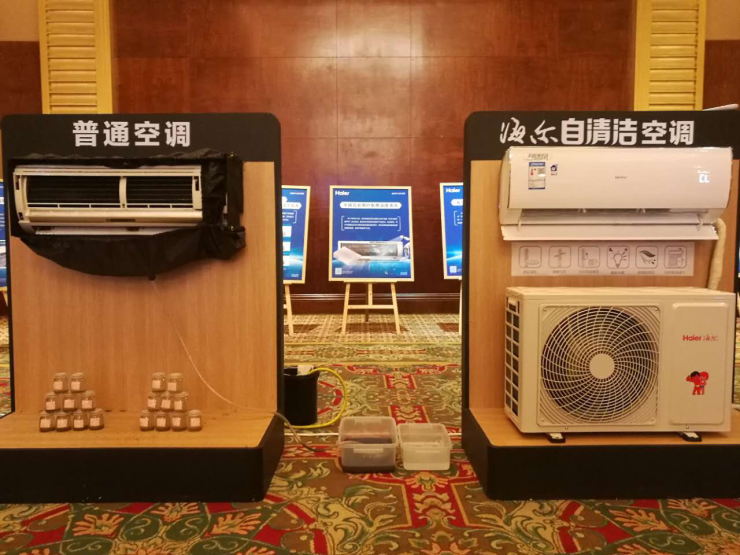|

# 智能自清洁空调白皮书:2年2倍速增长海尔第一

6月12日，中国家用电器商业协会、中怡康时代市场研究有限公司和《空调销售》杂志在北京联合发布了中国空调行业首份《智能自清洁空调行业白皮书》（以下简称《白皮书》）。《白皮书》指出，从2015年开始，智能自清洁空调的销售总量2年增长了2倍。自海尔首创智能自清洁空调后，各大品牌相继推出带有自动清洗功能的空调。从品牌占有率来看，中国自清洁空调市场品牌格局清晰，海尔等行业主流品牌在智能清洁空调市场的总体份额接近九成。海尔这两年更是全力主推，占据超过七成的份额，海尔智能自清洁空调零售量和零售额市场占比排名均是第一。

据介绍，今年3月10日到4月25日，《白皮书》项目组开展为期一个半月的市场调查，共收到有效问卷7943份。调查问卷反映了当下自清洁空调市场的情况，成为起草《白皮书》的重要依据之一。《白皮书》从消费趋势、市场格局、产品品类、规模化等方面具体阐释了截止到2017年4月自清洁空调的整体表现。空调消费升级自清洁成趋势

《白皮书》提到，我国正在进行第三次消费升级，其主要特征是消费需求细化和多样化。在空调行业则表现为制冷、制热的基础性能被智能、健康、舒适的体验替代。数据显示，2016年，智能空调占比18.2%，成为继变频空调之后，我国家用空调行业发展最快的产品类别。作为智能自清洁空调第一大品类，智能自清洁带来的健康空气解决方案成为用户刚需。在智能自清洁空调市场调查中发现，空调清洗难是困扰消费者的第一难题，还有超过3成的用户从不清洗空调。今年5月中旬，海尔启动空调免费深度清洗活动，20天时间从7000台空调中就洗出了44.8吨黑水。按照一个家庭平均每日160升用水量计算，这相当于一个家庭280天的用水量。目前，全国空调保有量超过5亿台，平均清洗一台空调耗水6.4升，以此计算，将会用去320万吨水，按照昆明湖200万立方米容积计算，相当于约1.6个昆明湖。2015年，海尔首创智能自清洁空调并快速推向市场，中怡康零售监测数据显示，2017年五一期间，自清洁空调在线上市场的零售量、零售额同比增速分别为408.9%、242.5%。自清洁空调成为影响消费者购买的重要因素之一。

自清洁市场格局清晰海尔占比第一

在智能自清洁空调成为行业新风口后，国内各大品牌相继推出带有自动清洗功能的空调。尤其是2017年前四个月，自清洁空调市场品牌快速增加，但总体市场格局清晰，海尔等行业主流品牌在智能自清洁空调市场的总体份额接近九成。

在自清洁市场主流品牌中，海尔智能自清洁占比七成，销量突破400万套，成为行业销售总量和增幅最大的单品。国家信息中心发布的数据显示，基于自清洁热销，海尔智能空调连续近40个月销量第一。

智能自清洁成智能第一大品类

现阶段，智能空调的主要功能集中包括WiFi手机控制、电费显示、语音交互、场景模拟等，而能吹洁净空气的智能自清洁空调不仅成为智能空调的第一大品类，而且成为智能空调普及以来第一款刚需单品。

从普通空调运行原理来看，空调使用一段时间后，由于反复空气循环，蒸发器翘片表面就会积聚大量灰尘、污垢及有害细菌。空调积累灰尘等物后，还会导致制冷、制热效果下降，增加耗电量和噪音。在此基础上，海尔智能自清洁空调等能自己洗澡的的产品被用户广泛认可。“蒸发器自清洁功能”也被首次列入智能空调的一项重要测评标准。

中怡康数据显示，自2016年1月开始，不论是自清洁空调还是智能自清洁空调，整体销售都出现稳步上升趋势。在智能市场占比中，智能自清洁空调零售量占比达到24.5%，销售额占比达到28.2%。自清洁进入全面普及时代

通过2年的市场积累，智能自清洁空调已经逐渐被消费者认可，特别是以海尔智能自清洁空调代表的健康空气解决方案成为市场竞争最大优势。数据显示，智能自清洁空调自诞生以来，销售总量以2倍以上的增长，开始进入规模化阶段。《白皮书》分析，自清洁空调的推出和普及，不仅关系到家庭健康生活方式的引领，而且关系到整个空调产业在智能时代转型升级的方向。目前，行业正在加速普及智能自清洁空调，例如，海尔智能自清洁空调实现了由“直播洗澡”到“吹白毛巾”再到“1杯水”的交互升级迭代，提升消费者认可度。在三四级市场迎来家电下乡后首个换新契机后，未来三年左右时间在智能空调的销售总量中，智能自清洁空调占比将超过5成。

中国家电电器商业协会副理事长、秘书长连四清分析，智能自清洁空调作为中国空调企业变革的一个细分市场新风口，为整个行业的发展带来增长的动力和活力。在我国家用空调的产量已占到世界总产量的80%以上的大背景下，以自清洁为代表的智能空调成为推动“中国制造”向“中国创造”转变的重要手段。海尔作为家电行业最早探索和实践智慧家庭的品牌，将为智能空调业注入全新活力，带动空调行业的良性快速发展。

`声明：本文由入驻焦点开放平台的作者撰写，除焦点官方账号外，观点仅代表作者本人，不代表焦点立场错误信息举报电话： 400-099-0099，邮箱：jubao@vip.sohu.com，或点此进行意见反馈，或点此进行举报投诉。`A B C D E F G H J K L M N P Q R S T W X Y Z
A - B - C - D - E
• A
• 鞍山
• 安庆
• 安阳
• 安顺
• 安康
• 澳门
• B
• 北京
• 保定
• 包头
• 巴彦淖尔
• 本溪
• 蚌埠
• 亳州
• 滨州
• 北海
• 百色
• 巴中
• 毕节
• 保山
• 宝鸡
• 白银
• 巴州
• C
• 承德
• 沧州
• 长治
• 赤峰
• 朝阳
• 长春
• 常州
• 滁州
• 池州
• 长沙
• 常德
• 郴州
• 潮州
• 崇左
• 重庆
• 成都
• 楚雄
• 昌都
• 慈溪
• 常熟
• D
• 大同
• 大连
• 丹东
• 大庆
• 东营
• 德州
• 东莞
• 德阳
• 达州
• 大理
• 德宏
• 定西
• 儋州
• 东平
• E
• 鄂尔多斯
• 鄂州
• 恩施
F - G - H - I - J
• F
• 抚顺
• 阜新
• 阜阳
• 福州
• 抚州
• 佛山
• 防城港
• G
• 赣州
• 广州
• 桂林
• 贵港
• 广元
• 广安
• 贵阳
• 固原
• H
• 邯郸
• 衡水
• 呼和浩特
• 呼伦贝尔
• 葫芦岛
• 哈尔滨
• 黑河
• 淮安
• 杭州
• 湖州
• 合肥
• 淮南
• 淮北
• 黄山
• 菏泽
• 鹤壁
• 黄石
• 黄冈
• 衡阳
• 怀化
• 惠州
• 河源
• 贺州
• 河池
• 海口
• 红河
• 汉中
• 海东
• 怀来
• I
• J
• 晋中
• 锦州
• 吉林
• 鸡西
• 佳木斯
• 嘉兴
• 金华
• 景德镇
• 九江
• 吉安
• 济南
• 济宁
• 焦作
• 荆门
• 荆州
• 江门
• 揭阳
• 金昌
• 酒泉
• 嘉峪关
K - L - M - N - P
• K
• 开封
• 昆明
• 昆山
• L
• 廊坊
• 临汾
• 辽阳
• 连云港
• 丽水
• 六安
• 龙岩
• 莱芜
• 临沂
• 聊城
• 洛阳
• 漯河
• 娄底
• 柳州
• 来宾
• 泸州
• 乐山
• 六盘水
• 丽江
• 临沧
• 拉萨
• 林芝
• 兰州
• 陇南
• M
• 牡丹江
• 马鞍山
• 茂名
• 梅州
• 绵阳
• 眉山
• N
• 南京
• 南通
• 宁波
• 南平
• 宁德
• 南昌
• 南阳
• 南宁
• 内江
• 南充
• P
• 盘锦
• 莆田
• 平顶山
• 濮阳
• 攀枝花
• 普洱
• 平凉
Q - R - S - T - W
• Q
• 秦皇岛
• 齐齐哈尔
• 衢州
• 泉州
• 青岛
• 清远
• 钦州
• 黔南
• 曲靖
• 庆阳
• R
• 日照
• 日喀则
• S
• 石家庄
• 沈阳
• 双鸭山
• 绥化
• 上海
• 苏州
• 宿迁
• 绍兴
• 宿州
• 三明
• 上饶
• 三门峡
• 商丘
• 十堰
• 随州
• 邵阳
• 韶关
• 深圳
• 汕头
• 汕尾
• 三亚
• 三沙
• 遂宁
• 山南
• 商洛
• 石嘴山
• T
• 天津
• 唐山
• 太原
• 通辽
• 铁岭
• 泰州
• 台州
• 铜陵
• 泰安
• 铜仁
• 铜川
• 天水
• 天门
• W
• 乌海
• 乌兰察布
• 无锡
• 温州
• 芜湖
• 潍坊
• 威海
• 武汉
• 梧州
• 渭南
• 武威
• 吴忠
• 乌鲁木齐
X - Y - Z
• X
• 邢台
• 徐州
• 宣城
• 厦门
• 新乡
• 许昌
• 信阳
• 襄阳
• 孝感
• 咸宁
• 湘潭
• 湘西
• 西双版纳
• 西安
• 咸阳
• 西宁
• 仙桃
• 西昌
• Y
• 运城
• 营口
• 盐城
• 扬州
• 鹰潭
• 宜春
• 烟台
• 宜昌
• 岳阳
• 益阳
• 永州
• 阳江
• 云浮
• 玉林
• 宜宾
• 雅安
• 玉溪
• 延安
• 榆林
• 银川
• Z
• 张家口
• 镇江
• 舟山
• 漳州
• 淄博
• 枣庄
• 郑州
• 周口
• 驻马店
• 株洲
• 张家界
• 珠海
• 湛江
• 肇庆
• 中山
• 自贡
• 资阳
• 遵义
• 昭通
• 张掖
• 中卫

1室1厅1厨1卫1阳台

1
2
3
4
5

0
1
2

1

1

0
1
2
3报名成功，资料已提交审核A B C D E F G H J K L M N P Q R S T W X Y Z
A - B - C - D - E
• A
• 鞍山
• 安庆
• 安阳
• 安顺
• 安康
• 澳门
• B
• 北京
• 保定
• 包头
• 巴彦淖尔
• 本溪
• 蚌埠
• 亳州
• 滨州
• 北海
• 百色
• 巴中
• 毕节
• 保山
• 宝鸡
• 白银
• 巴州
• C
• 承德
• 沧州
• 长治
• 赤峰
• 朝阳
• 长春
• 常州
• 滁州
• 池州
• 长沙
• 常德
• 郴州
• 潮州
• 崇左
• 重庆
• 成都
• 楚雄
• 昌都
• 慈溪
• 常熟
• D
• 大同
• 大连
• 丹东
• 大庆
• 东营
• 德州
• 东莞
• 德阳
• 达州
• 大理
• 德宏
• 定西
• 儋州
• 东平
• E
• 鄂尔多斯
• 鄂州
• 恩施
F - G - H - I - J
• F
• 抚顺
• 阜新
• 阜阳
• 福州
• 抚州
• 佛山
• 防城港
• G
• 赣州
• 广州
• 桂林
• 贵港
• 广元
• 广安
• 贵阳
• 固原
• H
• 邯郸
• 衡水
• 呼和浩特
• 呼伦贝尔
• 葫芦岛
• 哈尔滨
• 黑河
• 淮安
• 杭州
• 湖州
• 合肥
• 淮南
• 淮北
• 黄山
• 菏泽
• 鹤壁
• 黄石
• 黄冈
• 衡阳
• 怀化
• 惠州
• 河源
• 贺州
• 河池
• 海口
• 红河
• 汉中
• 海东
• 怀来
• I
• J
• 晋中
• 锦州
• 吉林
• 鸡西
• 佳木斯
• 嘉兴
• 金华
• 景德镇
• 九江
• 吉安
• 济南
• 济宁
• 焦作
• 荆门
• 荆州
• 江门
• 揭阳
• 金昌
• 酒泉
• 嘉峪关
K - L - M - N - P
• K
• 开封
• 昆明
• 昆山
• L
• 廊坊
• 临汾
• 辽阳
• 连云港
• 丽水
• 六安
• 龙岩
• 莱芜
• 临沂
• 聊城
• 洛阳
• 漯河
• 娄底
• 柳州
• 来宾
• 泸州
• 乐山
• 六盘水
• 丽江
• 临沧
• 拉萨
• 林芝
• 兰州
• 陇南
• M
• 牡丹江
• 马鞍山
• 茂名
• 梅州
• 绵阳
• 眉山
• N
• 南京
• 南通
• 宁波
• 南平
• 宁德
• 南昌
• 南阳
• 南宁
• 内江
• 南充
• P
• 盘锦
• 莆田
• 平顶山
• 濮阳
• 攀枝花
• 普洱
• 平凉
Q - R - S - T - W
• Q
• 秦皇岛
• 齐齐哈尔
• 衢州
• 泉州
• 青岛
• 清远
• 钦州
• 黔南
• 曲靖
• 庆阳
• R
• 日照
• 日喀则
• S
• 石家庄
• 沈阳
• 双鸭山
• 绥化
• 上海
• 苏州
• 宿迁
• 绍兴
• 宿州
• 三明
• 上饶
• 三门峡
• 商丘
• 十堰
• 随州
• 邵阳
• 韶关
• 深圳
• 汕头
• 汕尾
• 三亚
• 三沙
• 遂宁
• 山南
• 商洛
• 石嘴山
• T
• 天津
• 唐山
• 太原
• 通辽
• 铁岭
• 泰州
• 台州
• 铜陵
• 泰安
• 铜仁
• 铜川
• 天水
• 天门
• W
• 乌海
• 乌兰察布
• 无锡
• 温州
• 芜湖
• 潍坊
• 威海
• 武汉
• 梧州
• 渭南
• 武威
• 吴忠
• 乌鲁木齐
X - Y - Z
• X
• 邢台
• 徐州
• 宣城
• 厦门
• 新乡
• 许昌
• 信阳
• 襄阳
• 孝感
• 咸宁
• 湘潭
• 湘西
• 西双版纳
• 西安
• 咸阳
• 西宁
• 仙桃
• 西昌
• Y
• 运城
• 营口
• 盐城
• 扬州
• 鹰潭
• 宜春
• 烟台
• 宜昌
• 岳阳
• 益阳
• 永州
• 阳江
• 云浮
• 玉林
• 宜宾
• 雅安
• 玉溪
• 延安
• 榆林
• 银川
• Z
• 张家口
• 镇江
• 舟山
• 漳州
• 淄博
• 枣庄
• 郑州
• 周口
• 驻马店
• 株洲
• 张家界
• 珠海
• 湛江
• 肇庆
• 中山
• 自贡
• 资阳
• 遵义
• 昭通
• 张掖
• 中卫• 手机• 分享
• 设计
免费设计
• 计算器
装修计算器
• 入驻
合作入驻
• 联系
联系我们
• 置顶
返回顶部